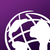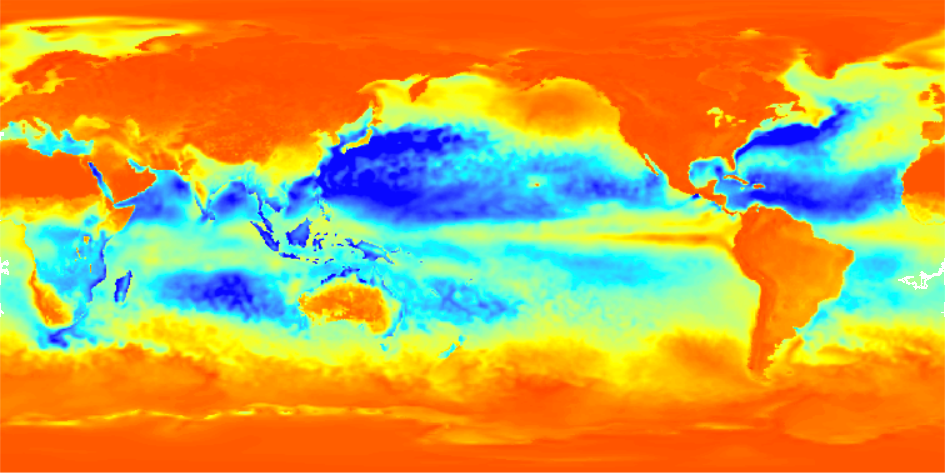# Export time dimension NetCDF to raster format using ArcPy

3749
24
06-11-2018 05:06 AMOccasional Contributor III

I have single NetCDF file which is time dimension NetCDF file, the netCDF file contains the monthly weather data for 20 years. I want to convert the NetCDF to raster tiff format and output of the raster as same the month and year name. below is code but it's producing the error in line number 29. Time format of the data is YY-MM-DD 2013-01-01 12:00, 2013-02-01 12:00, so on. How to loop all the time dimension.

I tried to extract the layer manually using ArcGIS tool, it's successfully extracted. I have copied the code from ArcGIS

``````arcpy.MakeNetCDFRasterLayer_md(in_netCDF_file="E:/Weather/2002.nc",
variable="slhf",
x_dimension="longitude",
y_dimension="latitude",
out_raster_layer="sshf_Layer",
band_dimension="",
dimension_values="time '01-01-2013 12:00:00'",
value_selection_method="BY_VALUE")‍‍‍‍‍‍‍‍‍‍‍‍‍‍‍‍‍‍‍‍‍‍‍‍‍‍‍‍‍‍‍‍``````
``````# Import system modules
import arcpy
from arcpy import env
from arcpy.sa import *

#Workspace
outLoc = r"E:\Weather/"
inNetCDF = r"E:\Weather\2002.nc"
#Veriable
variable = "slhf"
x_dimension = "lon"
y_dimension = "lat"
band_dimension = ""
dimension = "time"
valueSelectionMethod = "BY_VALUE"

nc_FP = arcpy.NetCDFFileProperties(inNetCDF)
nc_Dim = nc_FP.getDimensions()

for dimension in nc_Dim:
if dimension == "time":
top = nc_FP.getDimensionSize(dimension)
for i in range(0, top):

dimension_values = nc_FP.getDimensionValue(dimension, i)
nowFile = str(dimension_values)
nowFile = nowFile.translate(None, '/')
# I needed after 2013
if int(nowFile.split().split('-')[-1])==2013:

dv1 = ["time", dimension_values]
dimension_values = [dv1]

arcpy.MakeNetCDFRasterLayer_md(inNetCDF, variable, x_dimension, y_dimension, nowFile, band_dimension, dimension_values, valueSelectionMethod)
print "success"
outname = outLoc + nowFile

arcpy.CopyRaster_management(nowFile, outname,"", "", "", "NONE", "NONE", "")

else: print "DATA OUT OF RANGE"‍‍‍‍‍‍‍‍‍‍‍‍‍‍‍‍‍‍‍‍‍‍‍‍‍‍‍‍‍‍‍‍‍‍‍‍‍‍‍‍‍‍‍‍‍‍‍‍‍‍‍‍‍‍‍‍‍‍‍‍‍‍‍‍‍‍‍‍‍‍‍‍‍‍‍‍‍‍‍‍‍‍‍‍‍‍‍‍‍‍‍‍‍‍‍‍‍‍‍‍‍‍‍‍‍‍‍‍‍‍‍‍‍‍‍‍‍‍‍‍‍‍‍‍‍‍‍‍‍‍‍‍‍‍‍‍‍‍‍‍‍‍‍‍‍‍‍‍‍‍‍‍‍‍‍‍‍‍‍‍‍‍‍‍‍‍‍‍‍‍‍‍‍‍‍‍‍‍‍‍‍‍‍‍‍‍‍‍‍‍‍‍‍‍‍‍‍‍‍‍‍‍‍‍‍‍‍‍‍‍‍‍‍‍‍‍‍‍‍‍‍‍‍‍‍‍‍‍‍‍‍‍‍‍‍‍‍‍‍‍‍‍‍‍‍‍‍‍‍‍‍‍‍‍‍‍‍‍‍‍‍‍‍‍‍‍‍‍‍‍‍‍‍‍‍‍‍‍‍‍‍‍‍‍‍‍‍``````

Error message

``````ExecuteError: Failed to execute. Parameters are not valid.
ERROR 000237: One or more dimensions are invalid
ERROR 000237: One or more dimensions are invalid
Failed to execute (MakeNetCDFRasterLayer).‍‍‍‍‍‍‍‍‍‍‍‍‍‍‍‍‍‍‍‍‍‍‍‍‍‍‍‍‍‍‍‍``````
Tags (3)
1 Solution

Accepted SolutionsbyEsri Esteemed Contributor

Could you try the code below (changing the paths to the input NC file and output products)?

``````def main():
# Import system modules
import arcpy
import os
arcpy.env.overwriteOutput = True

#Workspace
outLoc = r"C:\GeoNet\NetCDF"
inNetCDF = r"C:\GeoNet\NetCDF\2013.nc"
#Veriable
variable = "slhf"
x_dimension = "longitude"
y_dimension = "latitude"
band_dimension = ""
dimension = "time"
valueSelectionMethod = "BY_VALUE"

nc_FP = arcpy.NetCDFFileProperties(inNetCDF)
print "nc_FP:", nc_FP
nc_Dim = nc_FP.getDimensions()
print "nc_Dim:", nc_Dim

for dimension in nc_Dim:
print "dimension:", dimension
if dimension == "time":
top = nc_FP.getDimensionSize(dimension)
print "top:", top
for i in range(0, top):
print "i", i
dimension_values = nc_FP.getDimensionValue(dimension, i)
year = GetYear(dimension_values)
print "year:", year
name = GetNameOutputFile(dimension_values)
print "name:", name

if year == '2013':
dv1 = "time '{}'".format(dimension_values)
# dv1 = ["time", dimension_values]
# dimension_values = [dv1]
out_ras_lay = "sshf_{}".format(name)

arcpy.MakeNetCDFRasterLayer_md(inNetCDF, variable, x_dimension, y_dimension, out_ras_lay, band_dimension, dv1, valueSelectionMethod)
print "success"
out_name =  "{}.tif".format(out_ras_lay)
out_ras = os.path.join(outLoc, out_name)
print "out_name:", out_name
print "out_ras:", out_ras

arcpy.CopyRaster_management(out_ras_lay, out_ras,"", "", "", "NONE", "NONE", "")
print "CopyRaster OK..."

else:
print "DATA OUT OF RANGE"

def GetNameOutputFile(dimension_value):
dct_ampm = {'P.':'PM', 'A.':'AM', 'PM': 'PM', 'AM': 'AM'}
lst1 = dimension_value.split(' ')
if '/' in dimension_value:
lst2a = lst1.split('/')
lst2b = lst1.split(':')
lst2a = CorrectList(lst2a)
lst2b = CorrectList(lst2b)
if len(lst1) >= 3:
ampm = lst1.upper()
if ampm in dct_ampm:
ampm = dct_ampm[ampm]
else:
ampm = 'XX'
name = 'd{}{}{}_t{}{}{}'.format(lst2a, lst2a, lst2a, lst2b, lst2b, ampm)
else:
name = 'd{}{}{}_t{}{}'.format(lst2a, lst2a, lst2a, lst2b, lst2b)

elif '-' in dimension_value:
lst2a = lst1.split('-')
lst2b = lst1.split(':')
name = 'd{}{}{}_t{}{}'.format(lst2a, lst2a, lst2a, lst2b, lst2b)
else:
name = 'd18990101_t0000'
return name

def GetYear(dimension_value):
lst1 = dimension_value.split(' ')
if '/' in dimension_value:
year = lst1.split('/')
elif '-' in dimension_value:
year = lst1.split('-')
else:
year = '1899'
return year

def CorrectList(lst2a):
lst = []
for a in lst2a:
if len(a) == 1:
lst.append('0{}'.format(a))
else:
lst.append(a)
return lst

if __name__ == '__main__':
main()‍‍‍‍‍‍‍‍‍‍‍‍‍‍‍‍‍‍‍‍‍‍‍‍‍‍‍‍‍‍‍‍‍‍‍‍‍‍‍‍‍‍‍‍‍‍‍‍‍‍‍‍‍‍‍‍‍‍‍‍‍‍‍‍‍‍‍‍‍‍‍‍‍‍‍‍‍‍‍‍‍‍‍‍‍‍‍‍‍‍‍‍‍‍‍‍‍‍‍‍‍‍‍‍‍‍``````
24 RepliesbyMVP Legendary Contributor

/blogs/dan_patterson/2016/08/14/script-formatting It is hard to read and your for loops look they are suffering from a bad copy paste into the code formatter

What are your dimensions? Why not print them out since at least one of them is apparently wrong.Occasional Contributor III

i have updated the code formatting and chosen Python format.
Regarding dimension, I have time dimension NetCDF file, based on each time file i am trying to export it as a raster.byMVP Legendary Contributor

print the dimensions as you get them in and around line 17-18 since one of your dimensions is wrong.

your indentation is wrong... just changing the parser to Python after the fact won't correct the script, you have to recopy and repasteOccasional Contributor III

Dan Patterson‌, Thank you. I have added the print statement but the print statement now showing any dimension of NetCDF. I have tried to convert the single layer of NetCDF file to raster using ArcGIS, its successful run. I have copied the code from ArcGIS.

``arcpy.MakeNetCDFRasterLayer_md(in_netCDF_file="E:/Weather/2002.nc", variable="slhf", x_dimension="longitude", y_dimension="latitude", out_raster_layer="sshf_Layer", band_dimension="", dimension_values="time '01-01-2013 12:00:00'", value_selection_method="BY_VALUE")``

but i have huge layer in NetCDF file, how to loop through all layer and convert it to rasterbyMVP Legendary Contributor

Can you show the updated code with the print statements and what it is actually printing (not the 'success' print) since one of the dimension names is invalid if the error is still the same)

For instance

x_dimension = "lon"
y_dimension = "lat"

should have been longitude and latitude as in you correct run

also

dv1 = ["time", dimension_values]
dimension_values = [dv1]

why did you make this a list of a list ? since dv1 is already a listbyEsri Esteemed Contributor

I would really like to know what the variable "nowFile" is filled with (if it gets there). Adding some print statements would really help and sharing the complete error message (including the line numbers) would help to understand where things go wrong.

Is it possible to attach the nc file?Occasional Contributor IIIbyMVP Legendary Contributor

Can you run this from your IDE's command line as well to get a listing of the dimensions

``````import netCDF4

f = r"C:\path\your_netcdf.nc"  # change to match one of your nc files
dataset = netCDF4.Dataset(f)
dataset.dimensions

# ----- report the list that is returned‍‍‍‍‍‍‍‍``````byEsri Esteemed Contributor

I just changed some code and did a test and it does produce several rasters. See code below:

``````# Import system modules
import arcpy
import os

#Workspace
outLoc = r"C:\GeoNet\NetCDF"
inNetCDF = r"C:\GeoNet\NetCDF\2013.nc"
#Veriable
variable = "slhf"
x_dimension = "longitude"  # Changed!
y_dimension = "latitude"  # Changed!
band_dimension = ""
dimension = "time"
valueSelectionMethod = "BY_VALUE"

nc_FP = arcpy.NetCDFFileProperties(inNetCDF)
print "nc_FP:", nc_FP
nc_Dim = nc_FP.getDimensions()
print "nc_Dim:", nc_Dim

for dimension in nc_Dim:
print "dimension:", dimension
if dimension == "time":
top = nc_FP.getDimensionSize(dimension)
print "top:", top
for i in range(0, top):
print "i", i
dimension_values = nc_FP.getDimensionValue(dimension, i)
print "dimension_values", dimension_values
nowFile = str(dimension_values)
print "nowFile (1):", nowFile
nowFile = nowFile.translate(None, '/')
print "nowFile (2):", nowFile

# I needed after 2013
test = int(nowFile.split().split('-')[-1])
print "test", test
if int(nowFile.split().split('-')[-1])==4021900:  # 2013
dv1 = "time '{}'".format(dimension_values)
# dv1 = ["time", dimension_values]
# dimension_values = [dv1]
out_ras_lay = "sshf_Layer_{}".format(i)

arcpy.MakeNetCDFRasterLayer_md(inNetCDF, variable, x_dimension, y_dimension, out_ras_lay, band_dimension, dv1, valueSelectionMethod)
print "success"
out_name =  "{}.tif".format(out_ras_lay)
out_ras = os.path.join(outLoc, out_name)
print "out_name:", out_name
print "out_ras:", out_ras

arcpy.CopyRaster_management(out_ras_lay, out_ras,"", "", "", "NONE", "NONE", "")
print "CopyRaster OK..."

else:
print "DATA OUT OF RANGE"‍‍‍‍‍‍‍‍‍‍‍‍‍‍‍‍‍‍‍‍‍‍‍‍‍‍‍‍‍‍‍‍‍‍‍‍‍‍‍‍‍‍‍‍‍‍‍‍‍‍‍‍‍‍‍``````

A number of things that caused problems, for instance:

• dimensions X and Y did not have the correct names on lines 10 and 11
• On line 38 (in my code) the validation was against 2013, however the year of the dates in the provided NC file was 1900. It never reached the part that converts to raster
• The dimension values were not correctly formatted (I'm using line 39)
• I changed the output raster layer name to a different unique name, which does not generate problems
• I also changed the output rasters names to avoid problems.

A resulting raster (12 were created):